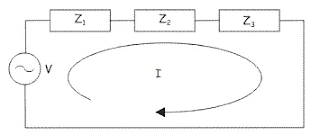### Circuit & Field Theory - short answer questions from AMIE exams (Summer 2019)

Explain the following in brief

Superposition theorem
Superposition theorem states that in any linear, bilateral network where more than one source is present, the response across any element in the circuit, is the sum of the responses obtained from each source considered separately while all other sources are replaced by their internal resistance. Superposition theorem is a circuit analysis theorem that is used to solve the network where two or more sources are present and connected.
The application of this theorem is both the AC & DC circuits where it assists to build the circuits like “Norton” as well as “Thevenin” equivalent circuits.

Norton’s theorem
Norton’s Theorem states that it is possible to simplify any linear circuit, no matter how complex, to an equivalent circuit with just a single current source and parallel resistance connected to a load.

Coulomb’s law
Coulomb’s Law.  Coulomb stated that the force between two very small objects separated in a vacuum by a distance that is large compared to their size is proportional to the charge on each and inversely proportional to the square of the distance between them, or

Where,
Q1, Q2   = positive or negative charges (C, Coulomb)
R = separation
ε= permittivity of free space( F/m, Farad/meter)

Biot-Savart’s law
Biot-Savart’s law is an equation that gives the magnetic field produced due to a current-carrying segment. This segment is taken as a vector quantity known as the current element. The Biot Savart law is fundamental to magnetostatics, playing a role similar to that of Coulomb’s law in electrostatics.

It is given by the following equation

Millman’s theorem
The Millman’s Theorem states that – when a number of voltage sources (V₁, V₂, V₃……… Vn) are in parallel having internal resistance (R₁, R₂, R₃………….Rn) respectively, the arrangement can replace by a single equivalent voltage source V in series with an equivalent series resistance R.
The utility of Millman’s Theorem is that the number of parallel voltage sources can be reduced to one equivalent source. It is applicable only to solve the parallel branch with one resistance connected to one voltage source or current source. It is also used in solving network having an unbalanced bridge circuit.

Equipotential surface
Electric potential is the potential energy per unit charge. Potential on an equipotential surface is constant through the surface. The direction of the electric field is perpendicular to the equipotential surface.

At every point on the equipotential surface, electric field lines are perpendicular to the surface. This is because potential gradient along any direction parallel to the surface is zero i.e.,
E = - (dv/dr) = 0
so component of electric Field parallel to equipotential surface is zero.

Open circuit natural frequency
An inductor stores energy as a magnetic field, a capacitor stores energy as an electric field. When an inductor and a capacitor are placed in series or parallel, they form a system that is equivalent to a weight on a spring.
Given an energy impulse (equivalent to pulling down the weight), energy is stored (whether the inductor is considered as the ‘spring’ or the ‘weight’ depends on whether we have a series or parallel circuit). When the impulse is removed, energy will shuttle back and forth between the two components, alternately stored as a magnetic and an electric field. It will do this at its natural frequency, given by

Dual network
Two electrical networks are said to be dual networks if the mesh equations of one network is equal to the node equation of the other. The dual network is based on Kirchhoff Current Law and Kirchhoff Voltage Law.
The networkV = I(Z1+Z2+Z3) [Mesh form]

Equation of this network in mesh form is
V = I(Z1+Z2+Z3) .... (1)

It is converted toV = I(Y1+Y2+Y3) [Nodal form]
Equation of this network in mesh form is
V = I(Y1+Y2+Y3) .... (2)
Here, the left side variable of equation (i) is voltage, and the left side variable of equation (ii) is current.
Similarly, the right side of equation (i) is a product of the current and total impedance of the circuit.
Similarly, the right side of equation (ii) is the product of voltage and admittance of the circuit.
So, it is needless to say these two networks are dual networks. From, the examples it is also clear that dual networks may not be equivalent networks.

The transfer function of a system
A transfer function is expressed as the ratio of the Laplace transform of output to the Laplace transform of input assuming all initial conditions to be zero.
Consider a system whose time-domain block diagram isTime-domain

where, r(t) = input and c(t) = output

Now taking Laplace transform thenLaplace domain

Now the transfer function G(s) is given by
G(s) = C(s)/R(s)

• The transfer function does not take into account the initial conditions.
• The transfer function can be defined for linear systems only.
• No inferences can be drawn about the physical structure of the system.
Displacement current
Displacement current is a quantity appearing in Maxwell’s Equation. It is defined in terms of the rate of change of the electric displacement field. The units of displacement current are the same as that of electric current density. Displacement current is not an electric current caused due to moving of charges(called conventional current), but it is caused by a time-varying electric field.
If we consider a capacitive circuit, we can see there is current flowing through the circuit. But if you look at the capacitor plates, there is a small empty region in between them.  Then how the circuit is completed?  The circuit is completed despite the small space because there is displacement in that region which is developed as a consequence of the electric field in between the plates.

---
The study material for AMIE/B Tech/Junior Engineer exams is available at https://amiestudycircle.com